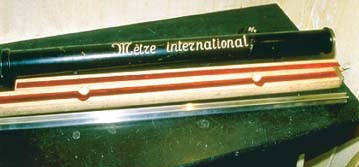What Is Distance?

This section introduces distance between two objects. The standard unit of distance is called 'meter', which is the length travelled by light in 1/299,792,458 of a second.

What Is Distance? Distance is a measurement that we use to describe how much space is there between two objects at a specific moment of time.

In order to measure distance, we have to define a standard unit. Currently (as of 1983), the standard unit to measure distance is called "meter" or "metre" defined by BIPM (Bureau International des Poids et Mesures) as: "the length of the path travelled by light in vacuum during a time interval of 1/299,792,458 of a second".

This definition sounds very precise. But it is based on two other factors:

• Unit of Time (Second) - We are tying the property, space, of our universe to another property, time.
• Speed of Light - We are assuming that the speed of light is a constant in this definition.

Before using the speed of light to define the "meter", CGPM (conférence générale des Poids et Mesures) used to construct a metal bar that has a length equal to a "meter", see the picture below. The length of that metal bar is defined as a meter.Standard Meter Bar to Measure Distance

With the standard unit defined, we can create measuring tapes and rulers that marked with multiples of meters and fractions within a meter. These tapes and rulers can then be used to measure distances between two objects as shown in the picture below: